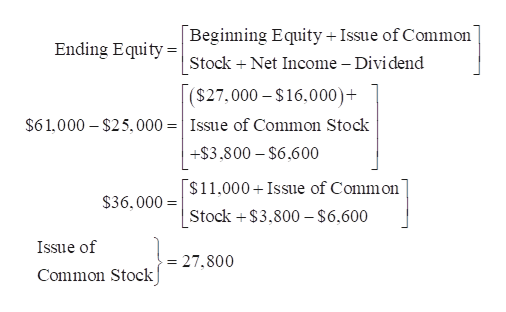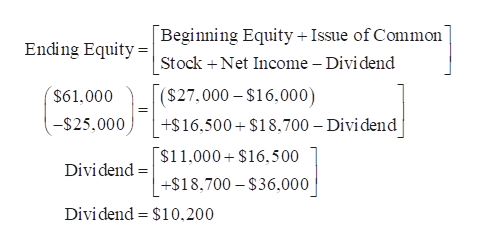# AssetsLiabilitiesBeginning of Year:\$27,000\$16,000End of Year:\$61,000\$25,0004) If net income is \$3,800 and dividends are \$6,600, how much is common stock?5) If the company issues common stock of \$16,500 and net income is \$18,700, how much is dividends? 6) If the company issues common stock of \$42,900 and pay dividends of \$3,100, how much is net income (loss)?

Question
2 views
Assets Liabilities
Beginning of Year: \$27,000 \$16,000
End of Year: \$61,000 \$25,000

4) If net income is \$3,800 and dividends are \$6,600, how much is common stock?

5) If the company issues common stock of \$16,500 and net income is \$18,700, how much is dividends?

6) If the company issues common stock of \$42,900 and pay dividends of \$3,100, how much is net income (loss)?

check_circle

Step 1

Compute the amount of common stock:help_outlineImage TranscriptioncloseBeginning Equity + Issue of Common Ending Equity = Stock + Net Income – Dividend (\$27.000 – \$16,000)+ \$61.000 – \$25,000 =| Issue of Common Stock +\$3,800 – \$6.600 \$11,000+ Issue of Common \$36,000 = Stock +\$3,800 – \$6,600 Issue of = 27,800 Common Stock fullscreen
Step 2

5. Compute the amount of dividend:...help_outlineImage TranscriptioncloseBeginning Equity + Issue of Common Ending Equity = Stock +Net Income – Dividend [(\$27.000 – \$16,000) \$61,000 -\$25,000 +\$16,500 + \$18,700 – Dividend \$11.000 + \$16,500 Dividend = +\$18,700 – \$36,000 Dividend = \$10,200 fullscreen

### Want to see the full answer?

See Solution

#### Want to see this answer and more?

Solutions are written by subject experts who are available 24/7. Questions are typically answered within 1 hour.*

See Solution
*Response times may vary by subject and question.
Tagged in